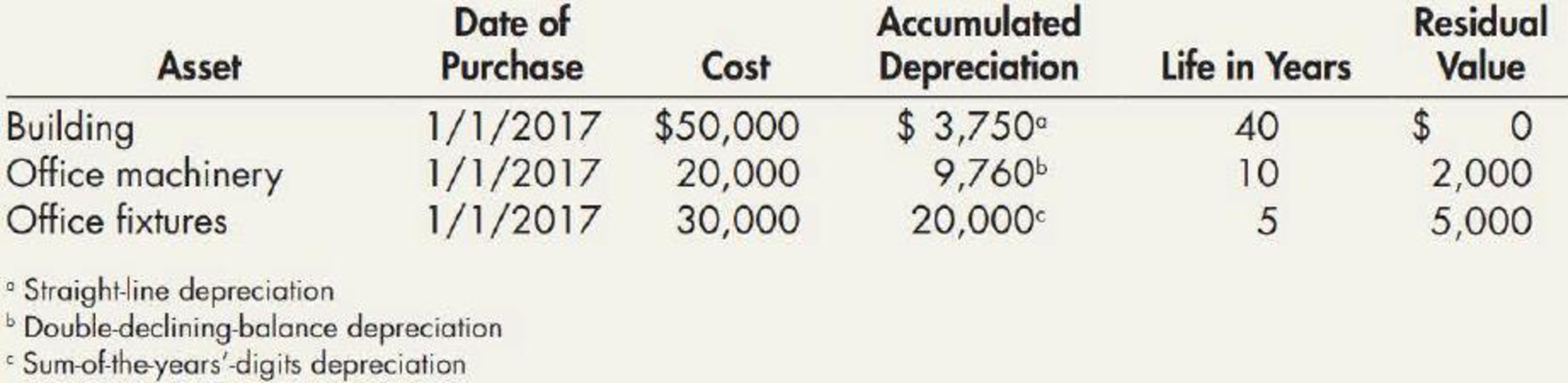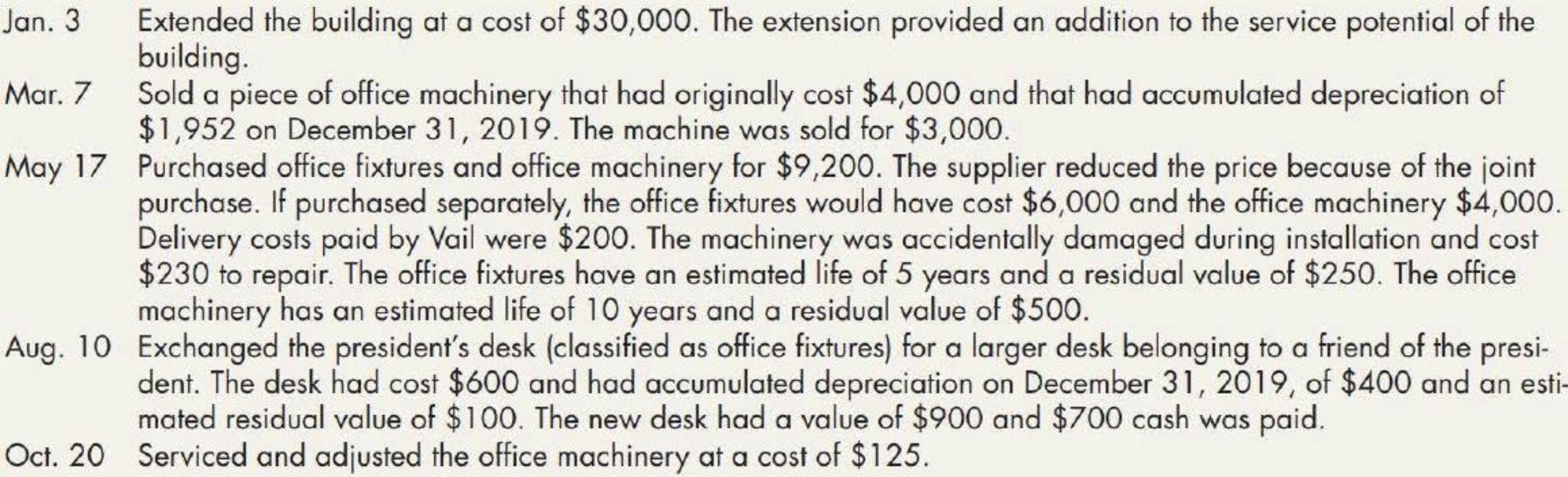Chapter 11, Problem 17P### Intermediate Accounting: Reporting...

3rd Edition
James M. Wahlen + 2 others
ISBN: 9781337788281

#### Solutions

Chapter
Section### Intermediate Accounting: Reporting...

3rd Edition
James M. Wahlen + 2 others
ISBN: 9781337788281
Textbook Problem
22 views

# On December 31, 2019, Vail Company owned the following assets:Vail computes depreciation and amortization expense to the nearest whole year. During 2020, Vail engaged in the following transactions:Required: 1. Check the accuracy of the accumulated depreciation balances at December 31, 2019. Round to the nearest whole dollar in all requirements. 2. Prepare journal entries to record the preceding events in 2020, as well as the year-end recording of depreciation expense. 3. Prepare an Accumulated Depreciation account for each category of assets, enter the beginning balance, post the journal entries from Requirement 2, and compute the ending balance.

1.

To determine

Calculate the accumulated depreciation balance at December 31, 2019 for the given assets, and check it’s ‘accuracy.

Explanation

Depreciation expense: Depreciation expense is a non-cash expense, which is recorded on the income statement reflecting the consumption of economic benefits of long-term asset on account of its wear and tear or obsolesces.

Straight-line depreciation method: The depreciation method which assumes that the consumption of economic benefits of long-term asset could be distributed equally throughout the useful life of the asset is referred to as straight-line method.

Sum-of- the-years’ digits method: Sum-of-the years’ digits method determines the depreciation by multiplying the depreciable base and declining fraction.

Double-declining-balance method: The depreciation method which assumes that the consumption of economic benefits of long-term asset is high in the early years but gradually declines towards the end of its useful life is referred to as double-declining-balance method.

Calculate the accumulated depreciation balance at December 31, 2019 for the given assets, and cross check its’ accuracy as follows:

Building:

Accumulated depreciation = (Annual depreciation expense×Number of years from 2017 to 2019)=$1,250(1)×3 years=$3,750

Office machinery:

 Year Beginning book value (A) Depreciation rate (2) (B) Depreciation expense C = A×B Ending book value D = A−C 2017 20,000 20% 4,000 16,000 2018 16,000 20% 3,200 12,800 2019 12,800 20% 2,560 10,240 Total 9,760

Table (1)

Note: Ending book value of the prior year is considered as the beginning book value of current year

2.

To determine

Prepare necessary journal entries for the given transaction for 2020.

3.

To determine

Prepare T-account for the accumulated depreciation, and calculate the ending balance of accumulated depreciation for the given assets.

### Still sussing out bartleby?

Check out a sample textbook solution.

See a sample solution

#### The Solution to Your Study Problems

Bartleby provides explanations to thousands of textbook problems written by our experts, many with advanced degrees!

Get Started

#### MIRR Refer to problem 11-1. What is the projects MIRR?

Fundamentals of Financial Management, Concise Edition (MindTap Course List)

#### Why should policymakers care about GDP?

Brief Principles of Macroeconomics (MindTap Course List)

#### Describe the two spans of management.

Foundations of Business (MindTap Course List)

#### CAPM AND REQUIRED RETURN Calculate the required rate of return for Manning Enterprises assuming that investors ...

Fundamentals of Financial Management, Concise Edition (with Thomson ONE - Business School Edition, 1 term (6 months) Printed Access Card) (MindTap Course List)